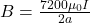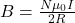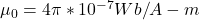## Two concentric circular coils of wire lie in a plane. The larger coil has N1 = 7200 turns and radius of a = 75.50 cm. The smaller coil has N

Question

Two concentric circular coils of wire lie in a plane. The larger coil has N1 = 7200 turns and radius of a = 75.50 cm. The smaller coil has N2 = 920 turns and radius of b = 1.00 cm. Ultimately we will find the Mutual Inductance, M, and then the induced emf, ϵmf. So let’s take it step by step.

First, what is the magnitude of the B-field at the smaller coil due to the larger coil?

in progress 0
7 months 2021-07-17T12:01:37+00:00 1 Answers 2 views 0

1. Note: The values of the current and the radii are not given, substitute whatever the value of the current and radius are to the given solution to obtain the magnitude.

magnitude of the B-field at the smaller coil due to the larger coil isExplanation:

N₁ = 7200 turns

N₂ = 920 turns

a = 75.50 cm = 0.755 m

b = 1.00 cm = 0.01 m

From the given data, b<<a, and it is at the center of the larger coil,

so we are safe to assume that the magnetic field at the smaller coil is constant.

The formula for magnetic field due to circular loop at the center of a coil is given by:Therefore, magnetic field in the smaller coil  due to the larger coil of radius a will be given by:WhereI = current in the larger coil